In this document, I show how to use some of the features of packages {bigsnpr} and {bigstatsr}. Note that many functions used here come from package {bigstatsr} and could therefore be used on other data encoded as matrix-like (outside of the field of genotype data).

## Get data

Download data and unzip files. I store those files in a directory called "tmp-data" here.

You can see there how I generated these data from the 1000 Genomes project.

## What you must do

You need to

• Explore the data

• Assess population structure of the data

• Find the variables associated with the disease status (y)

• Predict the disease status using the following indices as training/test sets:

# Divide the indices in training/test sets
set.seed(1)
ind.train <- sample(nrow(G), 400)
ind.test <- setdiff(rows_along(G), ind.train)

For this, you can use whatever tools you want because the data is quite small. In the following section, I give some (scalable) solutions using using packages {bigstatsr} and {bigsnpr}.

## Solution using {bigstatsr} and {bigsnpr}

### Population structure: Principal Component Analysis

Let us compute first principal components of the scaled genotype matrix:

# Compute partial SVD (10 PCs by default) using random projections
# big_scale() computes means and standard deviations for scaling
svd <- big_randomSVD(G, big_scale(), ncores = NCORES)
# Scree plot
plot(svd)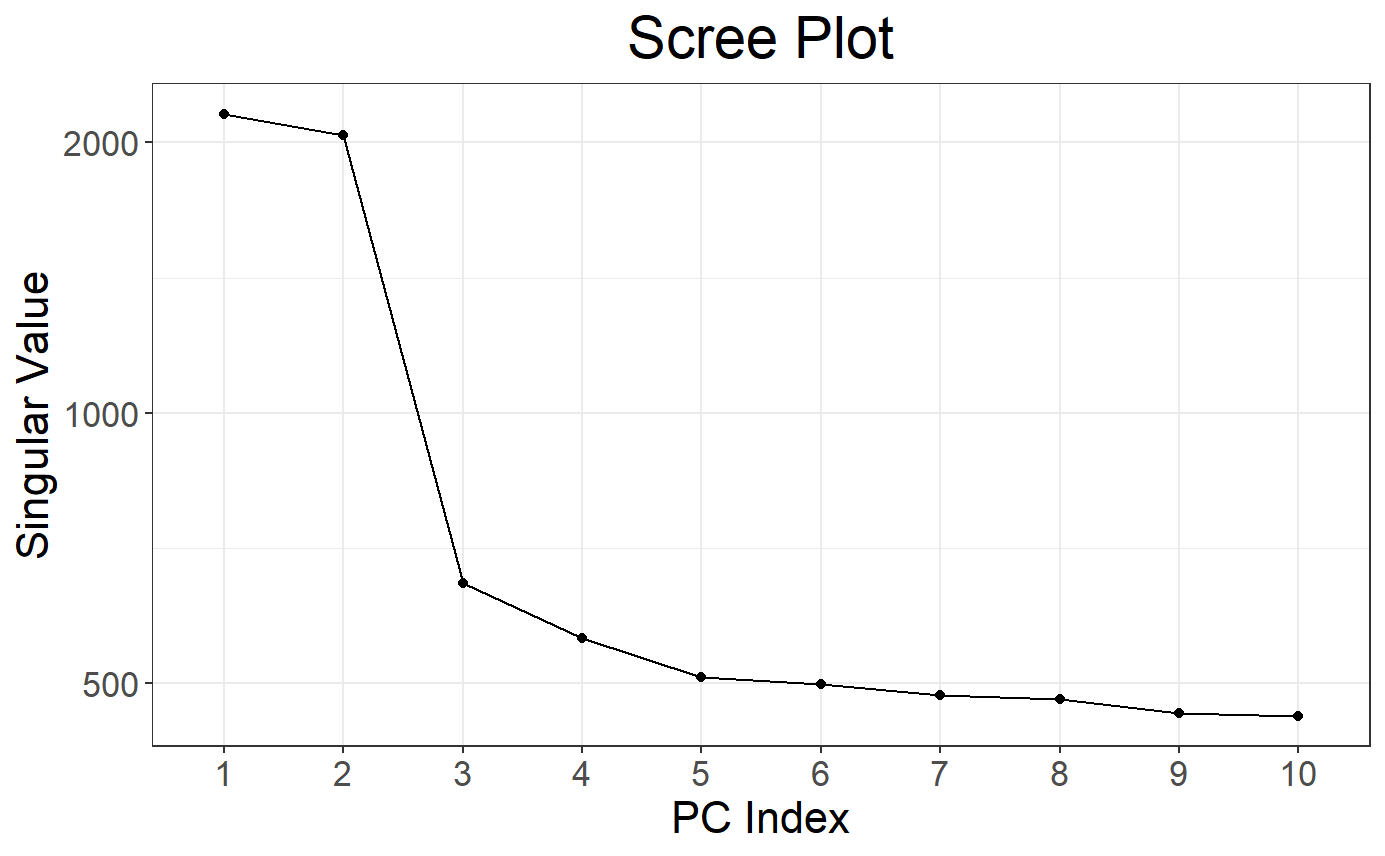library(ggplot2)
## Warning: package 'ggplot2' was built under R version 3.6.3
# Scores plot + color for population
plot(svd, type = "scores") +
aes(color = pop)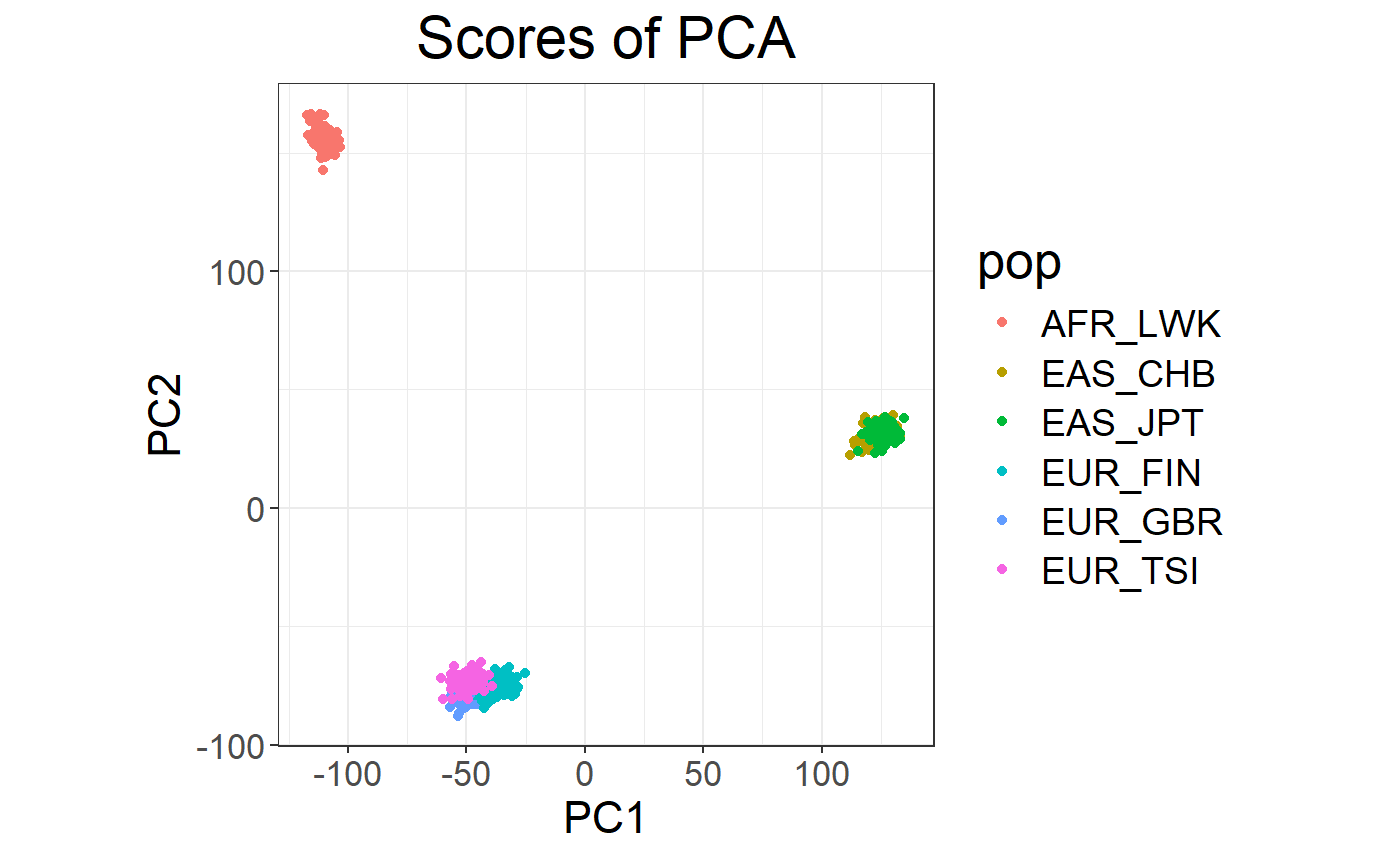plot(svd, type = "scores", scores = 3:4) +
aes(color = pop)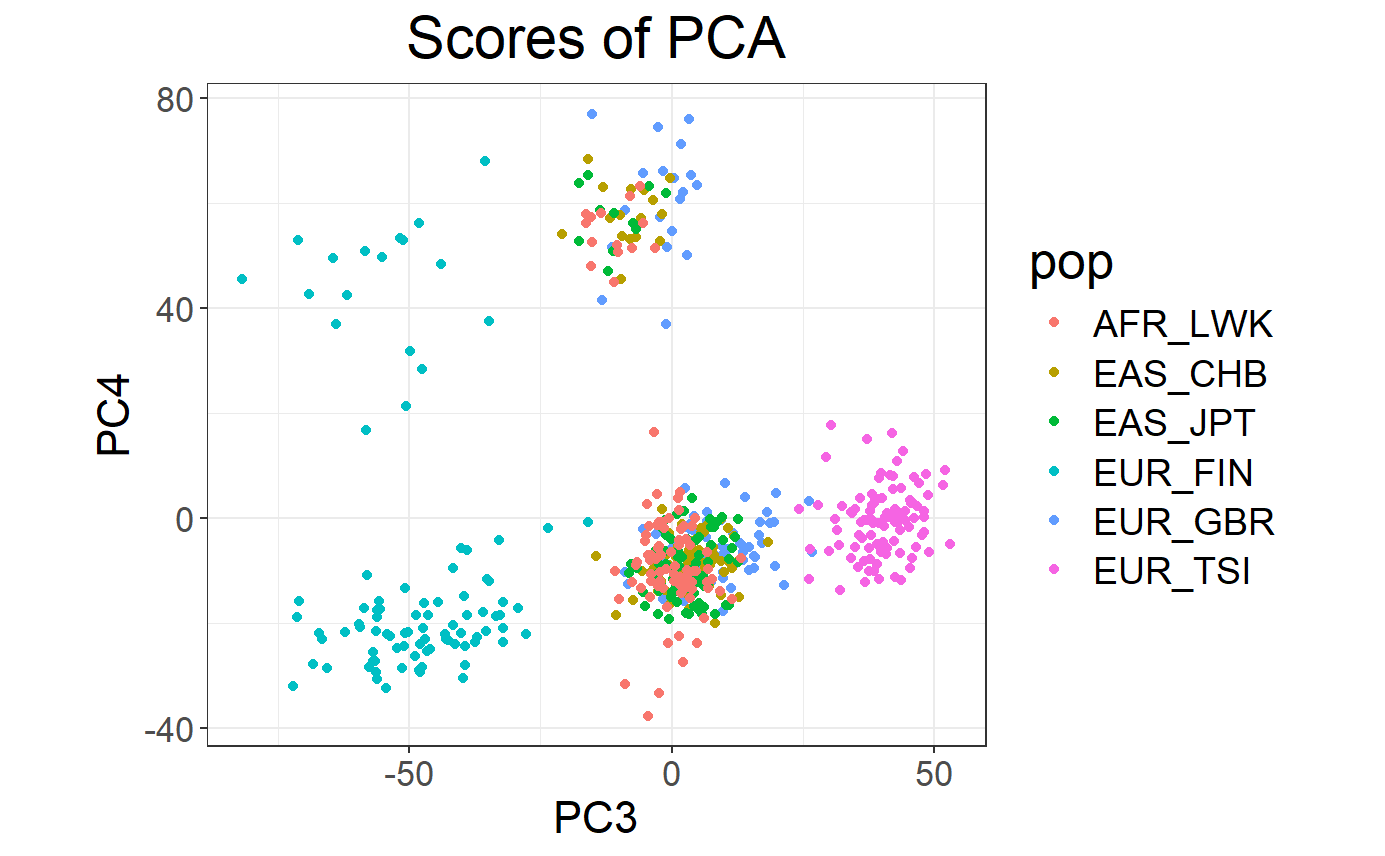# Loadings (effects of each variable for each PC)
plot(svd, type = "loadings", loadings = 1:10, coeff = 0.4)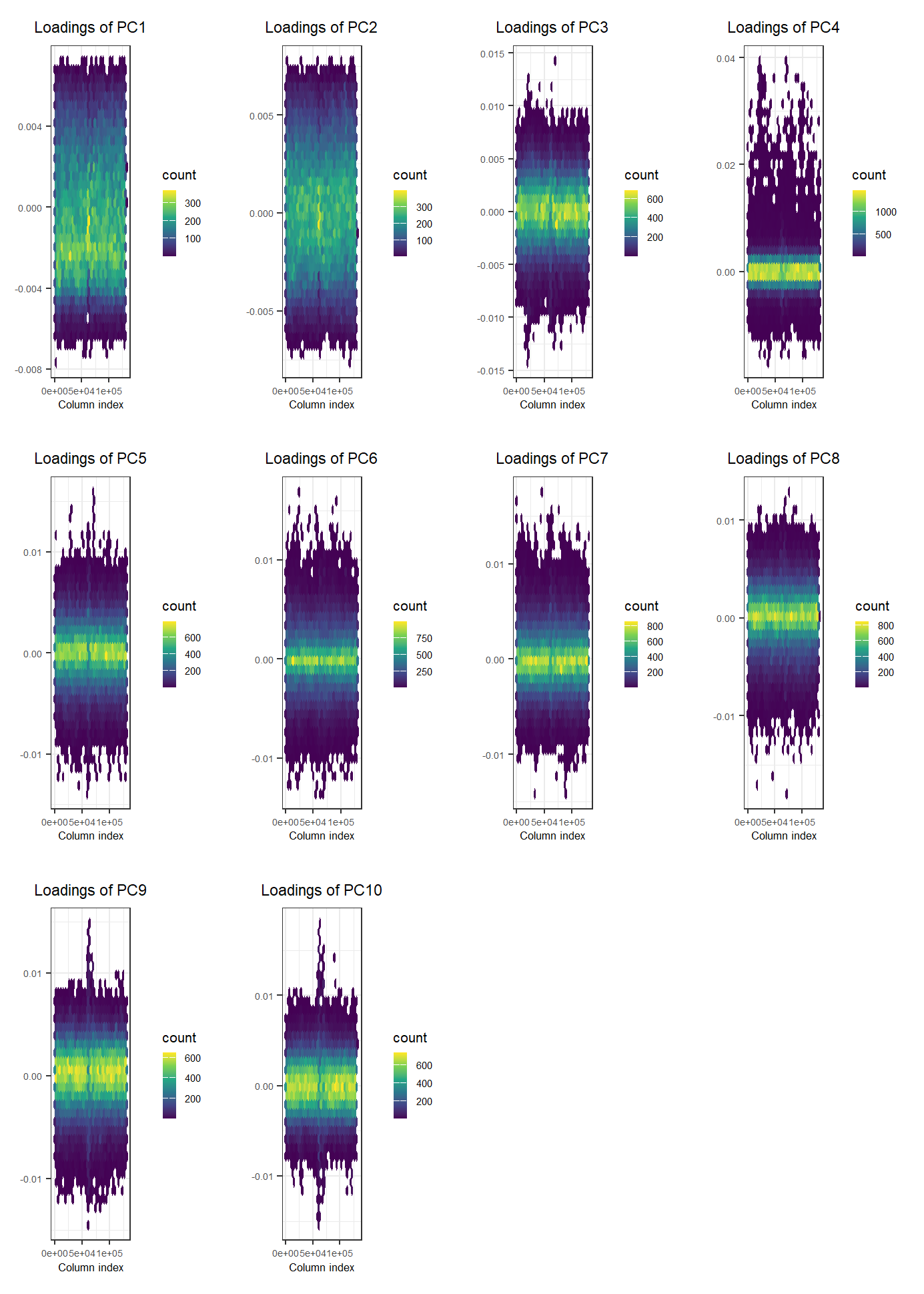### Association: Genome-Wide Association Study (GWAS)

# Association of each variable of G with y (adjusting for 10 PCs)
gwas <- big_univLogReg(G, y, covar.train = svd$u, ncores = NCORES) # Histogram of p-values plot(gwas)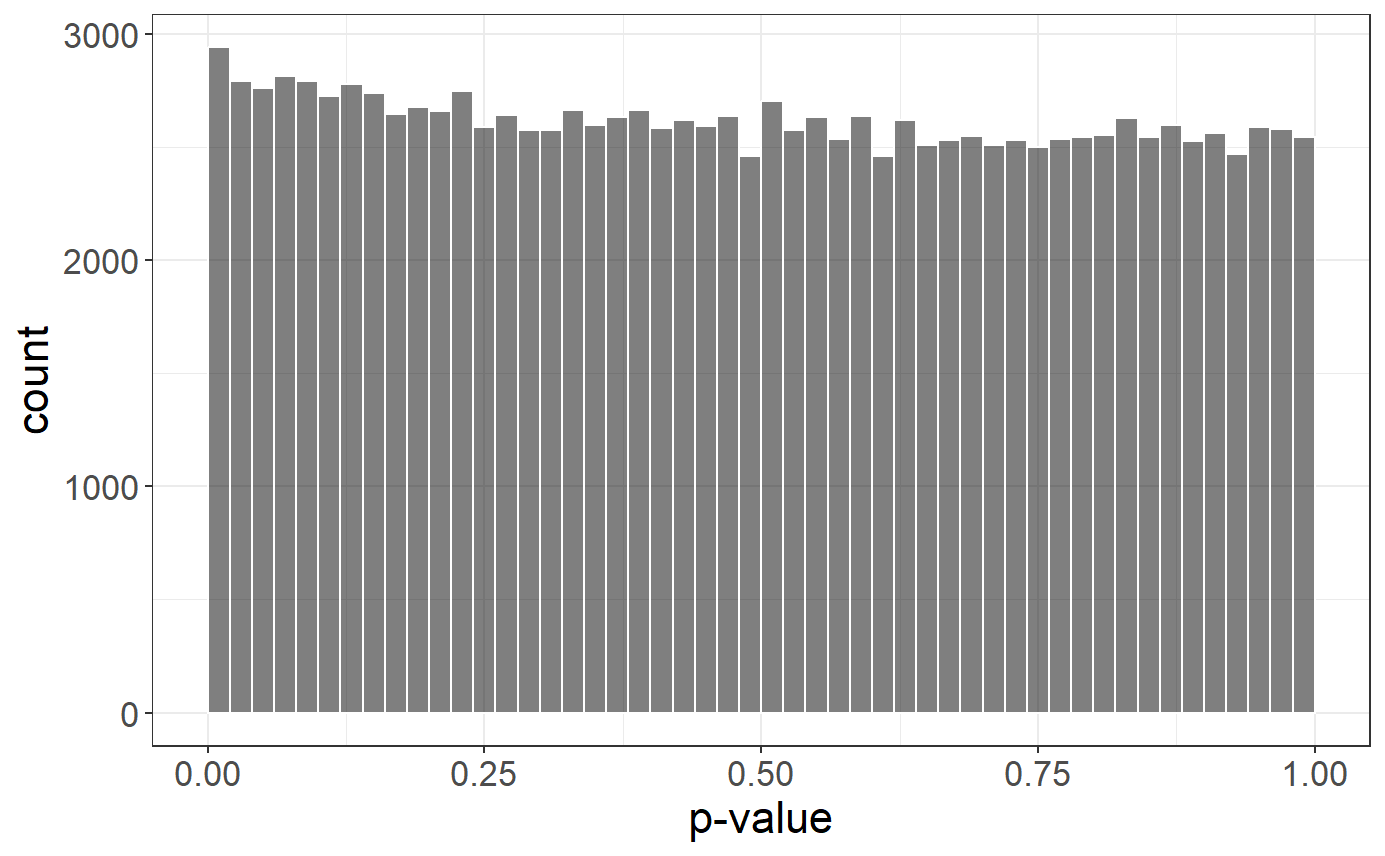# Q-Q plot plot(gwas, type = "Q-Q") + xlim(1, NA) # snp_qq(gwas) + xlim(1, NA) ## Warning: Removed 117734 rows containing missing values (geom_point).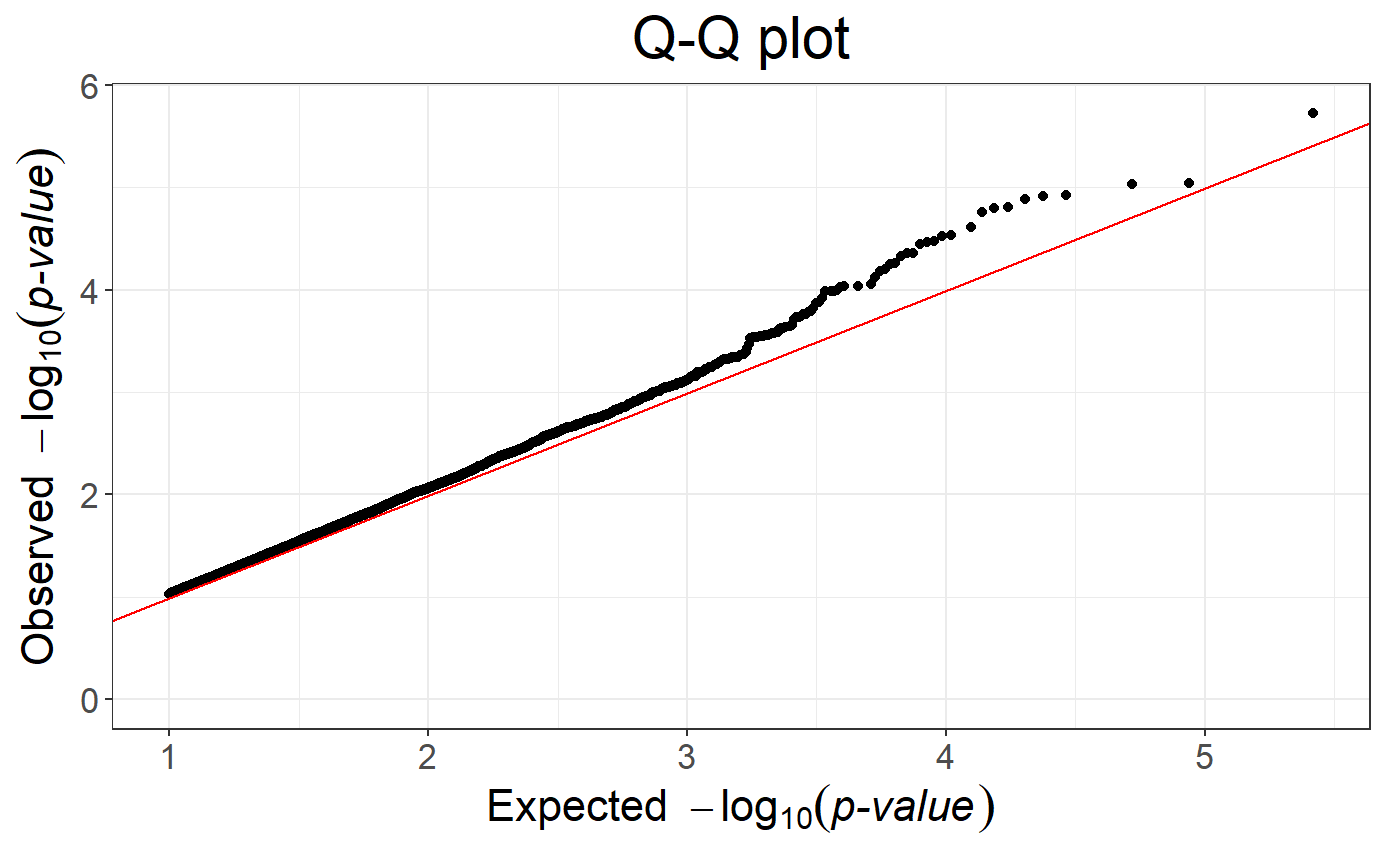# Manhattan plot snp_manhattan(gwas, CHR, POS, npoints = 20e3) + geom_hline(yintercept = -log10(5e-8), color = "red")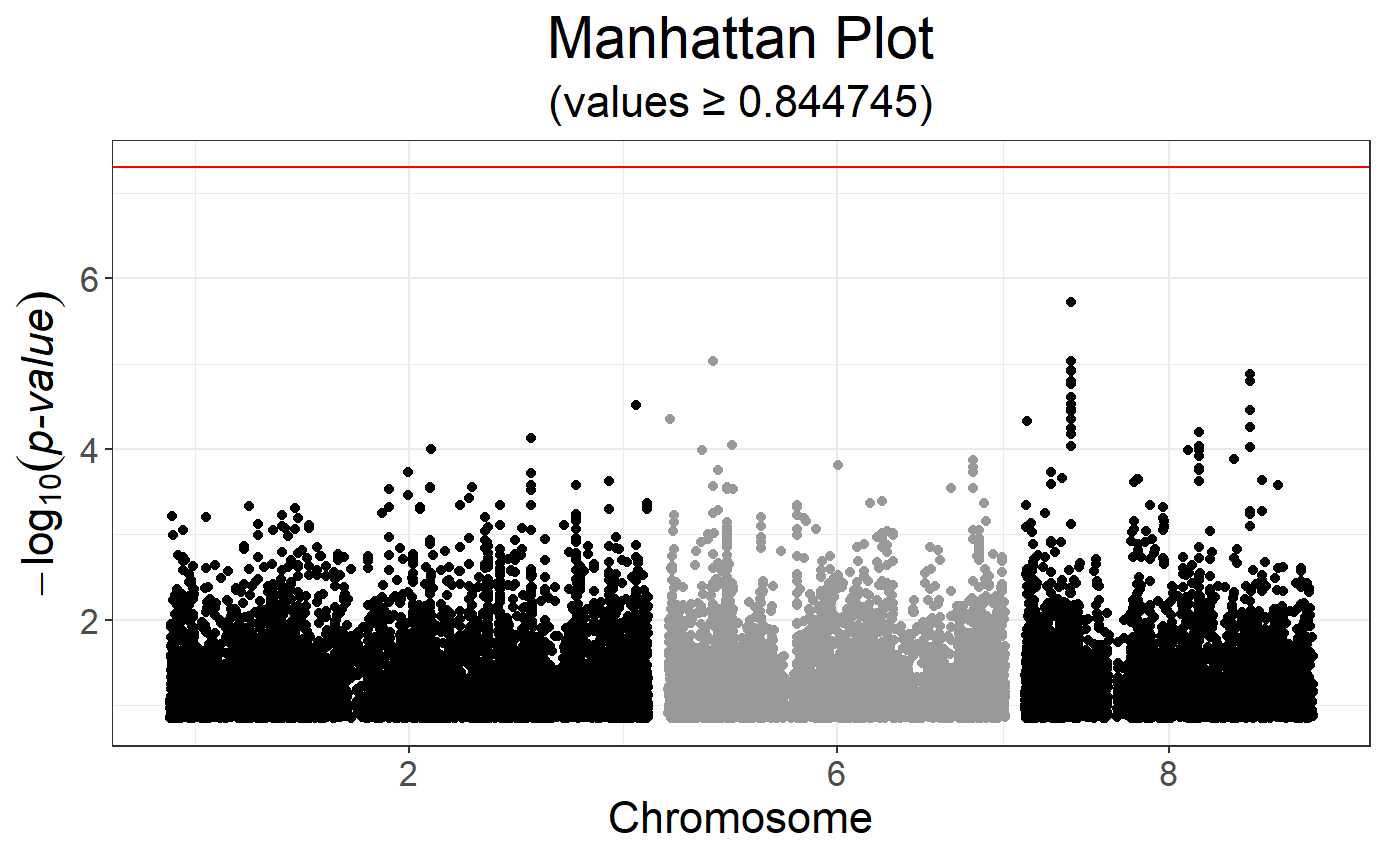### Polygenic Risk Score (PRS) #### with Clumping and Thresholding (C+T) $\rm{PRS}_i = \sum_{\substack{j \in S_\text{clumping} \\ p_j~<~p_T}} \hat\beta_j \cdot G_{i,j}~,$ where $$\hat\beta_j$$ ($$p_j$$) are the effect sizes (p-values) estimated from the GWAS and $$G_{i,j}$$ is the allele count (genotype) for individual $$i$$ and SNP $$j$$. sumstats <- bigreadr::fread2("tmp-data/public-data-sumstats.txt") lpval <- -log10(sumstats$p)
ind.keep <- snp_clumping(G, CHR, ind.row = ind.train, S = lpval, infos.pos = POS, ncores = 3)
THR <- seq_log(1, 8, length.out = 20)
prs <- snp_PRS(G, sumstats$beta[ind.keep], ind.keep = ind.keep, lpS.keep = lpval[ind.keep], thr.list = THR) # Learn the optimal threshold on the training set aucs <- apply(prs[ind.train, ], 2, AUC, target = y[ind.train]) plot(THR, aucs, xlab = "-log10(p-value)", ylab = "AUC", pch = 20)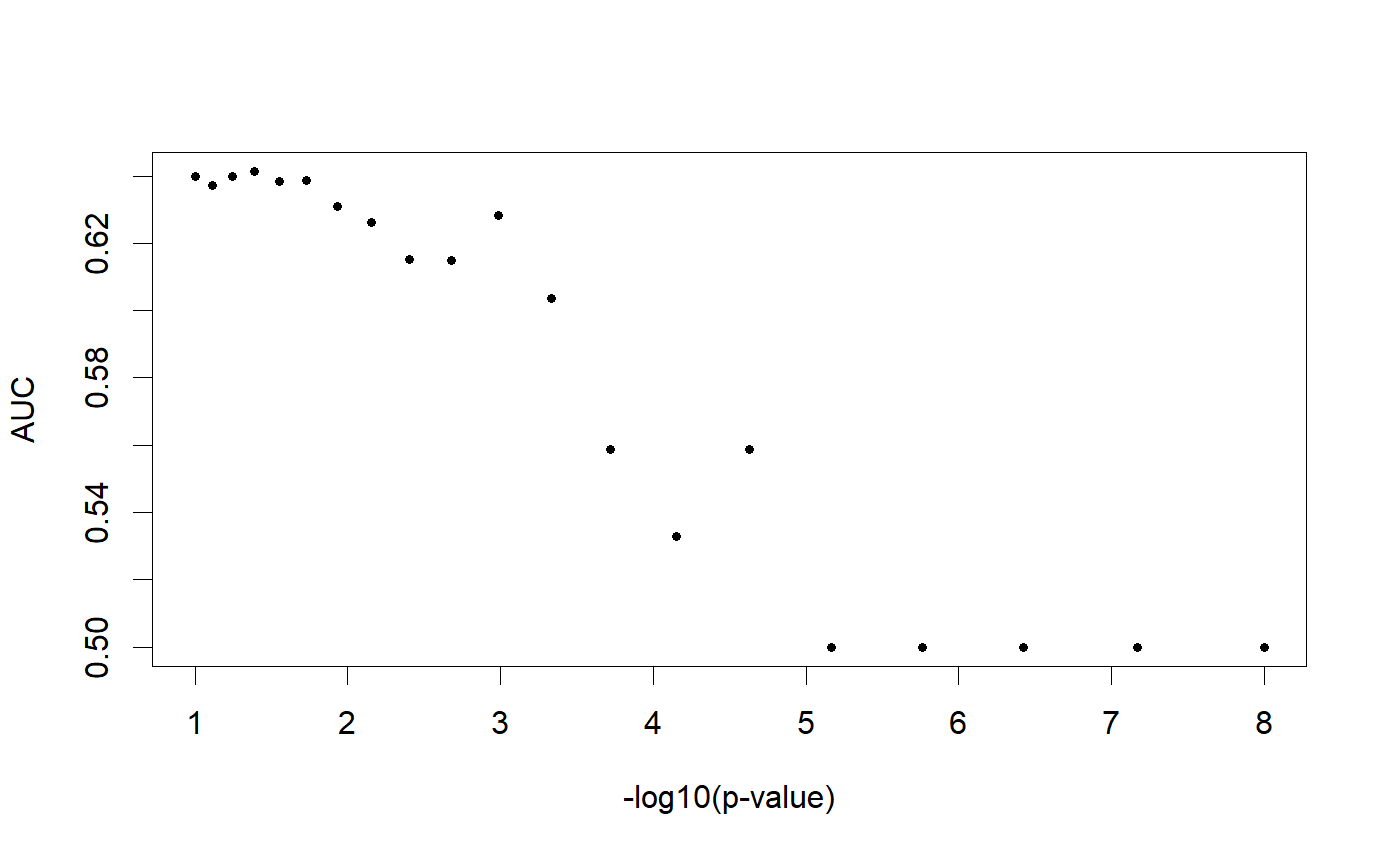# Evaluate on the test set AUC(prs[ind.test, which.max(aucs)], y[ind.test]) ##  0.6689111 (TODO: ADD SCT) #### with Penalized Logistic Regression (PLR) $\arg\!\min_{\beta_0,~\beta}(\lambda, \alpha)\left\{ \underbrace{ -\sum_{i=1}^n \left( y_i \log\left(p_i\right) + (1 - y_i) \log\left(1 - p_i\right) \right) }_\text{Loss function} + \underbrace{ \lambda \left((1-\alpha)\frac{1}{2}\|\beta\|_2^2 + \alpha \|\beta\|_1\right) }_\text{Penalization} \right\}$ where • $$p_i=1/\left(1+\exp\left(-(\beta_0 + x_i^T\beta)\right)\right)$$ • $$x$$ is denoting the genotypes and covariables (e.g. principal components), • $$y$$ is the disease status we want to predict, • $$\lambda$$ is a regularization parameter that needs to be determined and • $$\alpha$$ determines relative parts of the regularization $$0 \le \alpha \le 1$$. If you want to learn more about our implementation of PLR, please look at this paper. # Penalized logistic regression for many alphas and lambdas mod <- big_spLogReg(G, y[ind.train], ind.train, covar.train = svd$u[ind.train, ],
K = 5, alphas = 10^(-(0:4)), ncores = NCORES)
# Plot regularization paths (from high lambda to low lambda)
# for each validation set (color) and each alpha (facet)
plot(mod)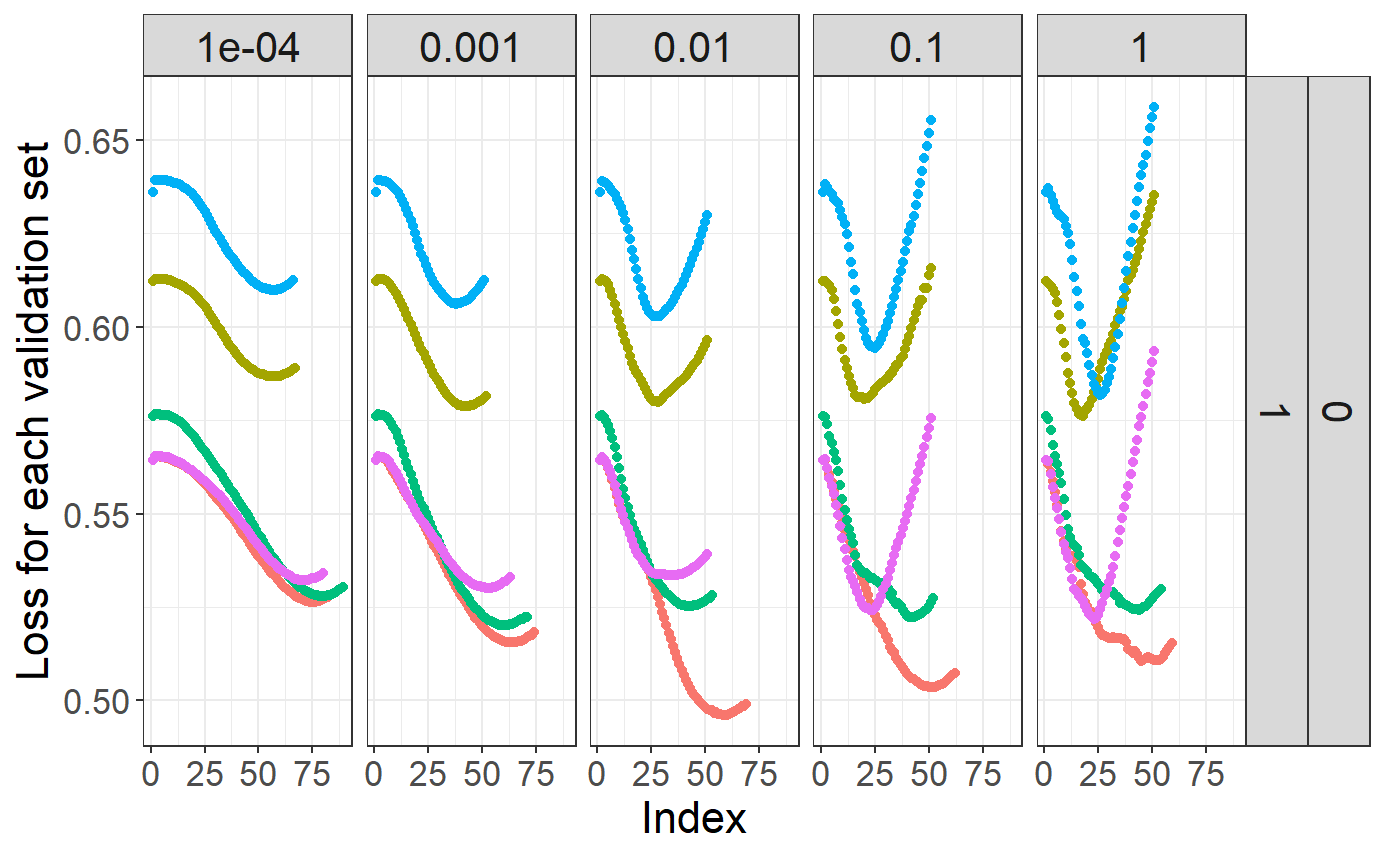# Get summaries of models
summary(mod)
## # A tibble: 5 x 9
##    alpha power_adaptive power_scale validation_loss intercept beta  nb_var
##    <dbl>          <dbl>       <dbl>           <dbl>     <dbl> <lis>  <int>
## 1 0.0001              0           1           0.557     -1.41 <dbl~  67876
## 2 0.001               0           1           0.550     -1.94 <dbl~  16008
## 3 0.01                0           1           0.547     -2.16 <dbl~   2928
## 4 0.1                 0           1           0.545     -2.30 <dbl~    722
## 5 1                   0           1           0.543     -2.40 <dbl~    398
## # ... with 2 more variables: message <list>, all_conv <lgl>
# Get the predictions for the test set
pred <- predict(mod, G, ind.test, covar.row = svd\$u[ind.test, ])
# Assess the Area Under the ROC Curve
AUC(pred, y[ind.test])
##  0.7148903